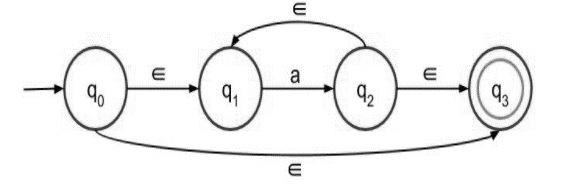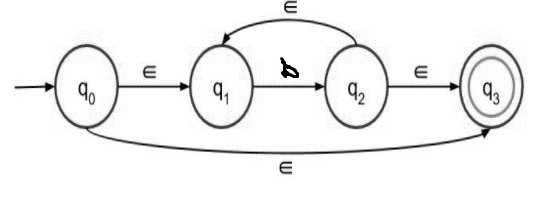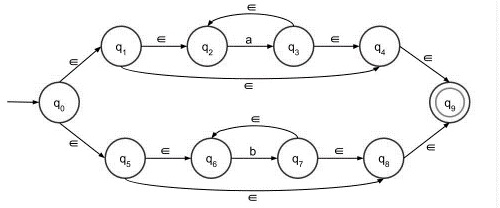# Construct a ‚àà-NFA for the language L = (a* + b*)

The ε transitions in Non-deterministic finite automata (NFA) are used to move from one state to another without having any symbol from input set Σ

ε-NFA is defined in five-tuple representation

{Q, q0, Σ, δ, F}

Where,

• δ − Q × (Σ∪ε)→2Q

• Q − Finite set of states

• Σ − Finite set of the input symbol

• q0 − Initial state

• F − Final state

• δ − Transition function

## NFA without ε transition

NFA is defined in five tuple representation

{Q, q0, Σ, δ, F}

Where,

• δ − Q X Σ→ 2Q

• Q − Finite set of states

• Σ − Finite set of the input symbol

• q0 − Initial state

• F − Final state

• δ − Transition function

NFA and NFA with epsilon both are almost the same; the only difference is their transition function.

Let’s consider the given language L = (a*+b*)

## Steps for construction ε-NFA

Step 1 − NFA with epsilon for a* is as follows −a* means there can be any number of ‘a’ in the expression, even 0 ( if the input symbol is null then also it is valid).

Step 2 − NFA with epsilon for b* is as follows −b* means there can be any number of b’s in the expression, even 0 (if input symbol is null then also it is valid).

Step 3 − Now construct a*+b* using first and second steps.

The given language is divided into two parts like a* and b* and add two steps by using ‘+’ sign to get the result.